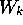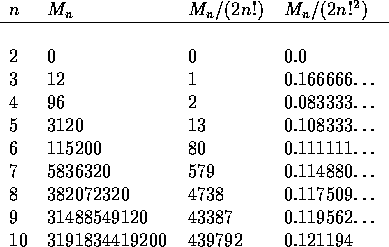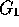# Non-sexist solution of the ménage problem

Kenneth P. Bogart
Peter G. Doyle

Last revised September 1985
Version 1.0A1 dated 5 September 1994

### Abstract:

The ménage problem asks for the number of ways of seating n couples at a circular table, with men and women alternating, so that no one sits next to his or her partner. We present a straight-forward solution to this problem. What distinguishes our approach is that we do not seat the ladies first.

# The ménage problem

The ménage problem (problème des ménages) asks for the numberof ways of seating n man-woman couples at a circular table, with men and women alternating, so that no one sits next to his or her partner. This famous problem was initially posed by Lucas  in 1891, though an equivalent problem had been raised earlier by Tait  in connection with his work on knot theory (see Kaplansky and Riordan ). This problem has been discussed by numerous authors (see the references listed in ), and many solutions have been found. Most of these solutions tell how to computeusing recurrence relations or generating functions, as opposed to giving an explicit formula. The first explicit formula for, was published by Touchard  in 1934, though he did not give a proof. Finally, in 1943, Kaplansky  gave a proof of Touchard's formula. Kaplansky's derivation was simple but not quite straight-forward, and the problem is still generally regarded to be tricky.

We will present a completely straight-forward derivation of Touchard's formula. Like Kaplansky's, our solution is based on the principle of inclusion and exclusion (see Ryser  and Riordan ). What distinguishes our approach is that we do not seat the ladies (or gentlemen) first.

# Solution to the relaxed ménage problem

We begin with an apparently simpler problem, called the relaxed ménage problem, which asks for the numberof ways of seating n couples around a circular table, so that no one sits next to his or her partner. This is nearly the same as the ménage problem, only now we have relaxed the requirement that men and women alternate.

To determine, we begin with the set S of all (2n)! ways of seating the 2n individuals around the table, and use inclusion-exclusion on the set of couples who end up sitting together. Let us call the elements of S seatings, and let us denote bythe number of seatings under which some specified set of k couples (and possibly some other couples) end up sitting together. Clearly,does not depend on the particular set of k couples we choose, and so, by the principle of inclusion and exclusion, we haveTo finish the enumeration, we must compute. Assume n>1. Letdenote the number of ways of placing k non-overlapping unlabeled dominos on 2n vertices arranged in a circle. (See Figure 1.)Figure 1: Non-overlapping dominos

Then(Decide where the k couples go, and which couple goes where, and which partner takes which seat, and where the 2n-2k individuals go.) So now we have only to compute the's. This is a routine combinatorial problem. The answer is(See Ryser , pp. 33-34, or Exercise 1 below). This yieldsPlugging this expression forinto the formula for, above, we getBy symmetry, we know that, must be divisible by. Pulling this factor out in front, we can writeThe first few values ofare shown in Table 1.Table 1: Relaxed ménage numbers

# Solution to the ménage problem

For the ménage problem, we proceed just as before, only now we restrict the set S of seatings to those where men and women alternate. The number of these seatings is: two ways to choose which seats are for men and which for women; n! ways to seat the men in the men's seats; n! ways to seat the women in the women's seats. Just as before, we havewheredenotes the number of alternating seatings under which a specified set of k couples all end up sitting together. This time we have(Decide which are men's seats and which women's, where the k couples go, which couple goes where, and where the n-k men and n-k women go.) Plugging in foryieldsPlugging this expression forinto the formula forabove, we getBy symmetry, we know thatmust be divisible by. Pulling this factor out in front, we can writeThe first few values ofare shown in Table 2.Table 2: Ménage numbers

# Comparison with Kaplansky's solution

The solution that we have just given is completely straight-forward and elementary, yet we have said that the ménage problem is still generally regarded to be tricky. How can this be? The answer can be given in two words: ``Ladies first.'' It apparently never occurred to anyone who looked at the problem not to seat the ladies first (or in a few cases, the gentlemen). Thus Kaplansky and Riordan 16] : ``We may begin by fixing the position of husbands or wives, say wives for courtesy's sake.''

Seating the ladies first ``reduces'' the ménage problem to a problem of permutations with restricted position. Unfortunately, this new problem is more difficult than the problem we began with, as we may judge from the cleverness of Kaplansky's solution :

We now restate the problème des ménages in the usual fashion by observing that the answer is, whereis the number of permutations ofwhich do not satisfy any of the following 2n conditions: 1 is 1st or 2nd, 2 is 2nd or 3rd,..., n is nth or 1st. Now let us select a subset of k conditions from the above 2n and inquire how many permutations ofthere are which satisfy all k; the answer is ( nk ) ! or 0 according as the k conditions are compatible or not. If we further denote bythe number of ways of selecting k compatible conditions from the 2n, we have, by the familiar argument of inclusion and exclusion,. It remains to evaluate, for which purpose we note that the 2n conditions, when arrayed in a circle, have the property that only consecutive ones are not compatible....

Of course, so we see how, by choosing to view the constraints as arrayed in a circle, Kaplansky has gotten back on the track of the straight-forward solution. We can only admire Kaplansky's cleverness in rediscovering the circle, and regret the tradition of seating the ladies first that made such cleverness necessary.

# Conclusion

It appears that it was only the tradition of seating the ladies first that made the ménage problem seem in any way difficult. We may speculate that, were it not for this tradition, it would not have taken half a century to discover Touchard's formula for. Of all the ways in which sexism has held back the advance of mathematics, this may well be the most peculiar. (But see Exercise 2.)

# Exercises

We list here, in the guise of exercises, some questions that you may want to explore with the help of the references listed.

1. Show how to ``derive'' the formula forsimply by writing down the answer, without using recurrence relations or generating functions or what have you. (Hint: Try this first for the formula for.)
2. Was it really sexism that made the ménage problem appear difficult? (See Kaplansky and Riordan , and the references listed there.)
3. Solve the analog of the ménage problem for the situation depicted in Figure 2. (No one is allowed to sit next to or across from his or her partner.)Figure 2: Real-world ménage problem.

4. Formulate the analog of the ménage problem for an arbitrary graph G, and show that it leads to a domino problem on G. Show that by seating the ladies or gentlemen first, and following Kaplansky's lead, we arrive at a problem of how to place rooks on a chessboard. (See Riordan , Ch. 7.) Show that the domino problem and the rook problem are equivalent. Look into the relationship of the domino problem to the Ising model of statistical mechanics. (See Fisher , Kasteleyn .)
5. What problem was Tait  really interested in? Did Gilbert  solve it? Show that Gilbert could have used a simple Möbius inversion argument instead of Pólya's theorem. What kinds of problems require the full force of Pólya's theorem?
6. What does it mean to ``solve'' a combinatorial problem like the ménage problem? Is a closed-form solution better than a recurrence? What if what we really want is to generate configurations, rather than just count them? (See Wilf .)
7. Why did Tait not pursue the ménage problem? What do knots have to do with atomic spectra? What was it like to live in Nebraska in the 1880's? (See Conway .)
8. The relaxed ménage problem can be further generalized as follows: Given two graphsandwith the same number of vertices, find the number of one-to-one mappings of the vertices ofonto the vertices ofsuch that no pair of vertices that are adjacent inget sent to vertices that are adjacent in. Show that the dinner table problem (see Aspvall and Liang , Robbins ) can be phrased in these terms, and give a solution using inclusion-exclusion. Formulate and solve an ``unrelaxed'' version of this problem. Show that the ménage problem can be phrased in these terms, and discuss how useful this reformulation is. Do the same for the problem of enumerating Latin rectangles (see Ryser  ).

## References

1
B. Aspvall and F. M. Liang. The dinner table problem. Technical Report STAN-CS-80-8222, Computer Science Department, Stanford University, Stanford, California, 1980.

2
J. H. Conway. An enumeration of knots and links, and some of their algebraic properties. In J. Leech, editor, Computational Problems in Abstract Algebra, pages 329-358. Pergamon, Oxford, 1970.

3
M. E. Fisher. Statistical mechanics of dimers on a plane lattice. Phys. Rev., 124:1664-1672, 1961.

4
E. N. Gilbert. Knots and classes of ménage permutations. Scripta Math., 22:228-233, 1956.

5
I. Kaplansky. Solution of the problème des ménages. Bull. Amer. Math. Soc., 49:784-785, 1943.

6
I. Kaplansky and J. Riordan. The problème des ménages. Scripta Mathematica, 12:113-124, 1946.

7
P. W. Kasteleyn. Dimer statistics and phase transitions. J. Math. Phys., 4:287-293, 1963.

8
E. Lucas. Théorie des nombres. Gauthier-Villars, Paris, 1891.

9
J. Riordan. An Introduction to Combinatorial Analysis. Wiley, New York, 1958.

10
D. Robbins. The probability that neighbors remain neighbors after random rearrangements. Amer. Math. Monthly, 87:122-124, 1980.

11
H. J. Ryser. Combinatorial Mathematics. Mathematical Association of America, Washington, D. C., 1963.

12
P. G. Tait. On knots, i, ii, iii. In Scientific Papers, pages 273-347. Cambridge Univ. Press, Cambridge, 1898.

13
J. Touchard. Sur un problème des permutations. C. R. Acad. Sciences Paris, 198:631-633, 1934.

14
H. Wilf. What is an answer? Amer. Math. Monthly, 89:289-292, 1982.

Peter Doyle